# Difference between revisions of "Arrangement"

with repetitions ofout ofelements

A finite sequence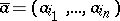of elements from some set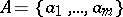. If all the term of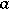are distinct, thenis called an arrangement without repetitions. The number of arrangements ofout ofelements with repetitions is, and that without repetitions in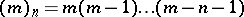.

An arrangement can be regarded as a functiongiven on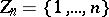and taking values in: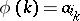,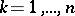. The elements ofare usually called cells (or urns), while those ofare called particles (or balls);defines the filling of the various cells by the various particles. If one speaks of indistinguishable particles or cells, it is understood that classes of arrangements are being considered. Thus, if all the particles are indistinguishable, then two arrangements defined by functionsand, respectively, belong to the same class if and only if there is a permutationof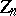such thatfor all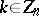. In this case, the number of such classes, or, as it is called, the number of arrangements fillingdifferent cells byindistinguishable particles, is the number of combinations ofout ofelements, with repetition allowed (cf. Combination).

If one says that all the cells are indistinguishable, then one means that the arrangements are put into the classes in such a way that two arrangements, defined by functionsandrespectively, belong to the same class if there exists a permutationoffor which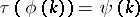for all. In this case the number of arrangements ofdifferent particles inindistinguishable cells, that is, the number of classes, is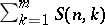, where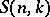are the Stirling numbers of the second kind: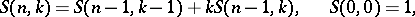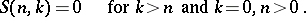If one does not distinguish either particles or cells, then an arrangement ofidentical particles inidentical cells is obtained; the number of such arrangements is, where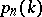is the number of additive partitions ofintonatural numbers. One can also consider other partitions of arrangements into classes, for example when the above-mentioned permutationsandare taken from subgroups of the symmetric groups of degreesand, respectively (this and other generalizations can be found in , ). Synonyms of "arrangement" are the terms "n-permutationn-permutation" , and "ordered n-sampleordered n-sample of a population" .

How to Cite This Entry:
Arrangement. Encyclopedia of Mathematics. URL: http://encyclopediaofmath.org/index.php?title=Arrangement&oldid=17266
This article was adapted from an original article by V.M. Mikheev (originator), which appeared in Encyclopedia of Mathematics - ISBN 1402006098. See original article